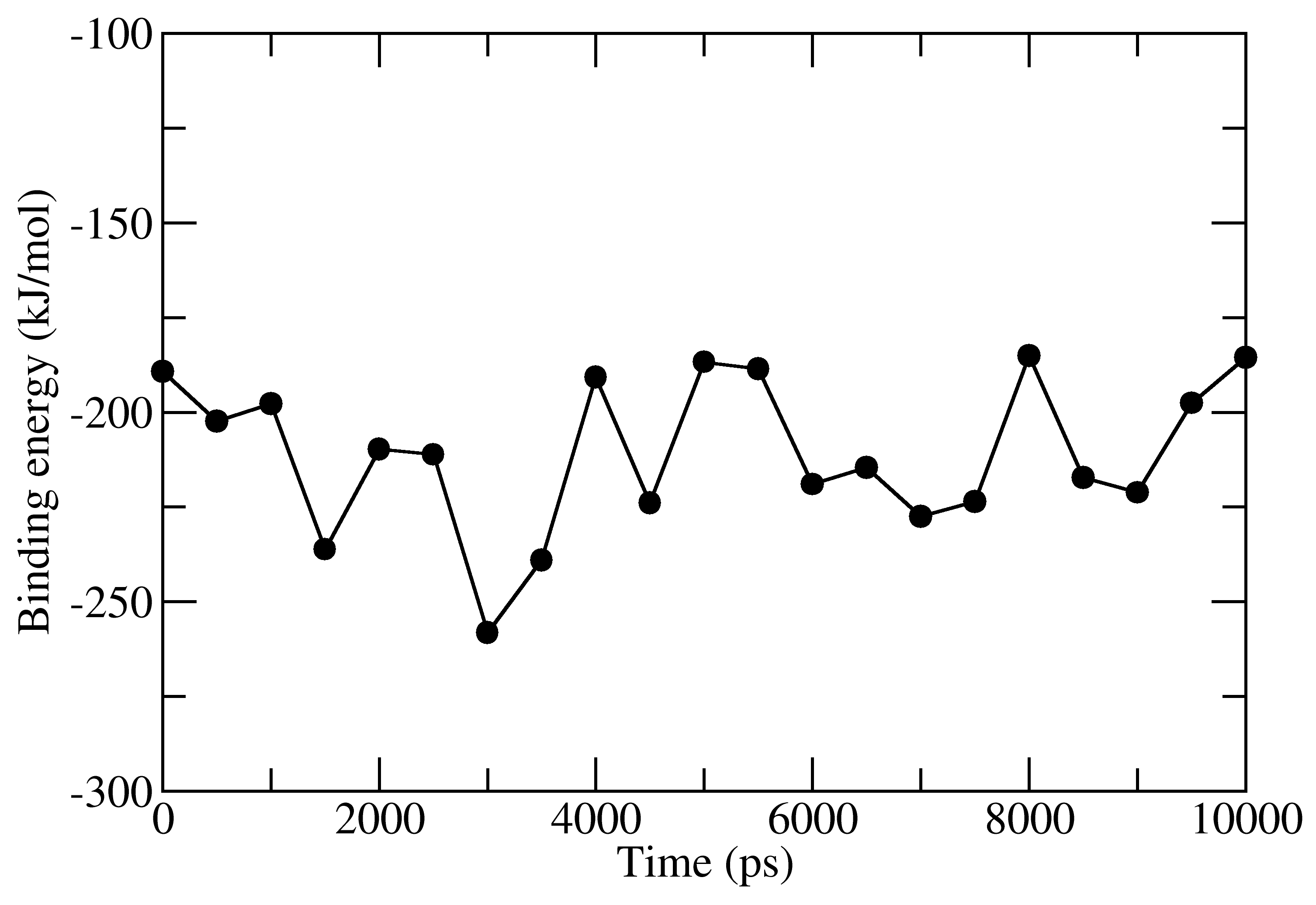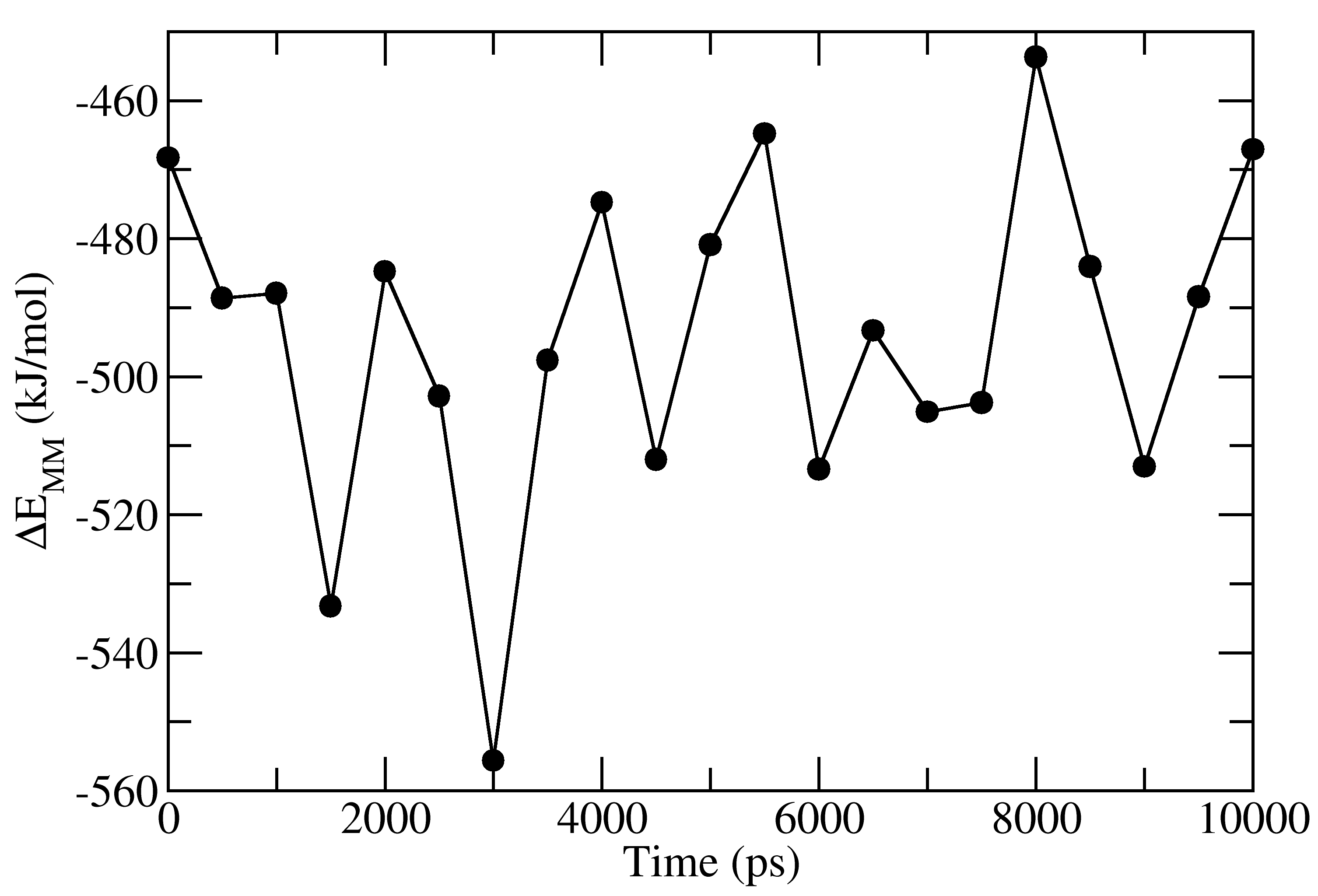# g_mmpbsa

MM/PBSA method for GROMACS.

This project is maintained by RashmiKumari

To download the tutorial package, click on this link. This package contains required file for the tutorial.

Untar this package by following command:

``````tar -zxvf tutorial.tar.gz
cd tutorial
cd 1EBZ
``````

This directory contains topology-parameter (tpr), atom-index (ndx), and trajectory (xtc) files of a HIV-1 protease inhibitor complex.

#### GMXLIB environment variable

If GROMACS is installed at custom location, set the GMXLIB environment variable:

``````export GMXLIB=/opt/gromacs/share/gromacs/top
``````

#### Calculation of three energy componenets

The binding energy consists of three energetic terms, (a) potential energy in vacuum, (b) polar-solvation energy and (c) non-polar solvation energy. These energetic terms could be calculated in either three or one step.

#### Three steps calculation

(a) Calculation of potential energy in Vacuum

Execute the following command, select 1 and 13 group number for protein and ligand, respectively:

``````g_mmpbsa -f 1EBZ.xtc -s 1EBZ.tpr -n 1EBZ.ndx -pdie 2 -decomp
``````

Two files `energy_MM.xvg` and `contrib_MM.dat` are generated as outputs. Both files could be generated with different name by `-mm filename1.xvg` and `-mmcon filename2.dat`. `energy_MM.xvg` file contains van der Waals, electrostatic interactions, and net non-bonded potential energy between the protein and inhibitor. `contrib_MM.dat` contains contribution of each residue to the calculated net non-bonded interaction energy.

(b) Calculation of polar solvation energy

To calculate the polar solvation energy, an input file (e.g. tutorial/polar.mdp) is required. This file contains input parameters that are used in the calculation of polar solvation energy. To get the details of the input parameters, please click on this link.

Execute the following command, select 1 and 13 group number for protein and ligand, respectively:

``````g_mmpbsa -f 1EBZ.xtc -s 1EBZ.tpr -n 1EBZ.ndx -i ../polar.mdp -nomme -pbsa -decomp
``````

Two files `polar.xvg` and `contrib_pol.dat` are generated as outputs. Both files could be generated with different name by `-pol filename1.xvg` and `-pcon filename2.dat`. `polar.xvg` contains polar solvation energies for unbound protein, unbound inhibitor and protein-inhibtor complex. `contrib_pol.dat` contains contribution of each residue to the calculated net polar solvation energy.

(c) Calculation of non-polar solvation energy

To calculate the non-polar solvation energy, an input file (e.g. tutorial/apolar_sasa.mdp) is required. This file contains parameters that are used in the calculation of non-polar solvation energy. To get the details of the input parameters, please click on this link. There are several type of non-polar models that are discussed in this publication (see Table 1 for values). Here, SASA-only and SAV-only model are used for which input parameter files are provided.

For SASA-only model:

Execute the following command, select 1 and 13 group number for protein and ligand, respectively:

g_mmpbsa -f 1EBZ.xtc -s 1EBZ.tpr -n 1EBZ.ndx -i ../apolar_sasa.mdp -nomme -pbsa -decomp -apol sasa.xvg -apcon sasa_contrib.dat`

Two files `sasa.xvg` and `sasa_contrib.dat` are generated as outputs. `sasa.xvg` contains non-polar solvation energy for unbound protein, unbound inhibitor and protein-inhibtor complex. `sasa_contrib.dat` contains contribution of each residue to the calculated net polar-solvation energy.

For SAV-only model:

Execute the following command, select 1 and 13 group number for protein and ligand, respectively:

g_mmpbsa -f 1EBZ.xtc -s 1EBZ.tpr -n 1EBZ.ndx -i ../apolar_sav.mdp -nomme -pbsa -decomp -apol sav.xvg -apcon sav_contrib.dat`

#### One step calculation

Execute the following command, select 1 and 13 group number for protein and ligand, respectively:

``````g_mmpbsa -f 1EBZ.xtc -s 1EBZ.tpr -n 1EBZ.ndx -i ../pbsa.mdp -pdie 2 -pbsa -decomp`
``````

`pbsa.mdp` contains input parameters for both polar and SASA-only non-polar solvation energies. All three energetic terms are calculated by using the above single command and all output files are generated.

#### Average Binding Energy Calculation

To calculate average binding energy, a python script MmPbSaStat.py is provided in the `g_mmpbsa` package. For details about this script, please click on this link. To execute this script, above obtained files are required as the inputs.

To calculate average binding energy by direct method, execute following command:

``````python MmPbSaStat.py -m energy_MM.xvg -p polar.xvg -a apolar.xvg (or sasa.xvg)
``````

Two output files `full_energy.dat` and `summary_energy.dat` are obtained. `summary_energy.dat` contains average and standard deviations of all energetic components including the binding energy as follows:

``````#Complex Number:    1
===============
SUMMARY
===============

van der Waal energy      =        -334.587   +/-   15.514 kJ/mol

Electrostattic energy    =        -159.380   +/-   15.810 kJ/mol

Polar solvation energy   =         313.698   +/-   10.174 kJ/mol

SASA energy              =         -30.431   +/-    0.996 kJ/mol

SAV energy               =           0.000   +/-    0.000 kJ/mol

WCA energy               =           0.000   +/-    0.000 kJ/mol

Binding energy           =        -210.699   +/-   19.745 kJ/mol

===============
END
===============
``````

`full_energy.dat` contains the values of energetic terms as a function of time. Last four columns contains Δ_E_MM, Δ_G_polar, Δ_G_nonpolar and Δ_G_binding as a function of time. These quantities could be plotted with xmgrace/matplotlib/gnuplot. The respective four files in xmgrace format (agr) are provided in `tutorial/1EBZ/output`To calculate average binding energy by using bootstrap analysis, execute following command:

``````python MmPbSaStat.py -bs -nbs 2000 -m energy_MM.xvg -p polar.xvg -a apolar.xvg (or sasa.xvg)
``````

Again, two output files `full_energy.dat` and `summary_energy.dat` are genrated as outputs. `full_energy.dat` is similar to that of the above one. However, `summary_energy.dat` contains average and standard error of all energetic components including the binding energy. Average values in `summary_energy.dat` are slightly different from the above one. For more details about this method, please follow this publication.